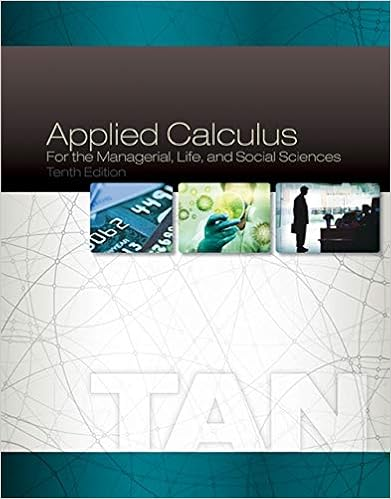# Select one a current ratio no impact working capital

• Test Prep
• 290
• 96% (67) 64 out of 67 people found this document helpful

This preview shows page 172 - 178 out of 290 pages.

##### We have textbook solutions for you!
The document you are viewing contains questions related to this textbook.The document you are viewing contains questions related to this textbook.
Chapter 6 / Exercise 41
Applied Calculus for the Managerial, Life, and Social Sciences
TanExpert Verified
current ratio and the working capital, respectively?Select one:a. Current ratio: no impactWorking capital: decrease b. Current ratio: increaseWorking capital: no impact c. Current ratio: no impactWorking capital: no impact d. Current ratio: increaseWorking capital: increase FeedbackYour answer is correct.The correct answer is: Current ratio: no impactWorking capital: no impact(T / F) The cost of land includes its purchase price and other related costs, including the cost of removing an old unusable building that is on the land.Select one:True False FeedbackCorrect.The cost of land includes all normal, reasonable, and necessary expenditures to obtain the land and get it ready for use.The correct answer is 'True'.Question 2
##### We have textbook solutions for you!
The document you are viewing contains questions related to this textbook.The document you are viewing contains questions related to this textbook.
Chapter 6 / Exercise 41
Applied Calculus for the Managerial, Life, and Social Sciences
TanExpert Verified
CorrectMark 1.00 out of 1.00Flag questionQuestion text(T / F) The formula for calculating straight-line depreciation is: Depreciation per period = (Asset cost – estimated salvage value) / Number of accounting periods (estimated useful life).Select one:True False FeedbackCorrect.The correct answer is 'True'.Question 3CorrectMark 1.00 out of 1.00Flag questionQuestion text(T / F) Units-of-production method: Assigns an equal amount of depreciation to each unit of product manufactured by an asset.Select one:True False Feedback
Correct.The correct answer is 'True'.Question 4CorrectMark 1.00 out of 1.00Flag questionQuestion text(T / F) Double-declining-balance method is an accelerated deprecation method. Salvage value is ignored in making annual calculations (save for the final depreciation period).Select one:True False FeedbackCorrect.The correct answer is 'True'.Question 5CorrectMark 1.00 out of 1.00Flag questionQuestion text(T / F) The formula for DDB deprecation is: Deprecation per period = (2×straight-line rate)×(Asset cost – Accumulated deprecation.)Select one:
True False FeedbackCorrect.The correct answer is 'True'.Question 6CorrectMark 1.00 out of 1.00Flag questionQuestion text(T / F) Expenditures that increase the quality of services or extend the quantity of services beyond the original estimate are capital expenditures.Select one:True False FeedbackCorrect.The correct answer is 'True'.Question 7CorrectMark 1.00 out of 1.00Flag question
Question text(T / F) In calculating depletion, the residual value of acquired land containing an ore deposit is included in total costs subject to depletion.Select one:True False FeedbackCorrect. The residual value of land should be deducted from total costs subject to depletion.The correct answer is 'False'.Question 8CorrectMark 1.00 out of 1.00Flag questionQuestion text(T / F) All recorded intangible assets are subject to amortization.Select one:True False FeedbackCorrect. Only intangible assets with finite useful lives should be amortized.The correct answer is 'False'.Question 9CorrectMark 1.00 out of 1.00
Flag questionQuestion text(T / F) By comparing an asset's book value (cost less up-to-date accumulated depreciation) with its sales price, the company may show either a gain or a loss.Select one:True False FeedbackCorrect.The correct answer is 'True'.Question 10CorrectMark 1.00 out of 1.00Flag questionQuestion text
•••